# 比较器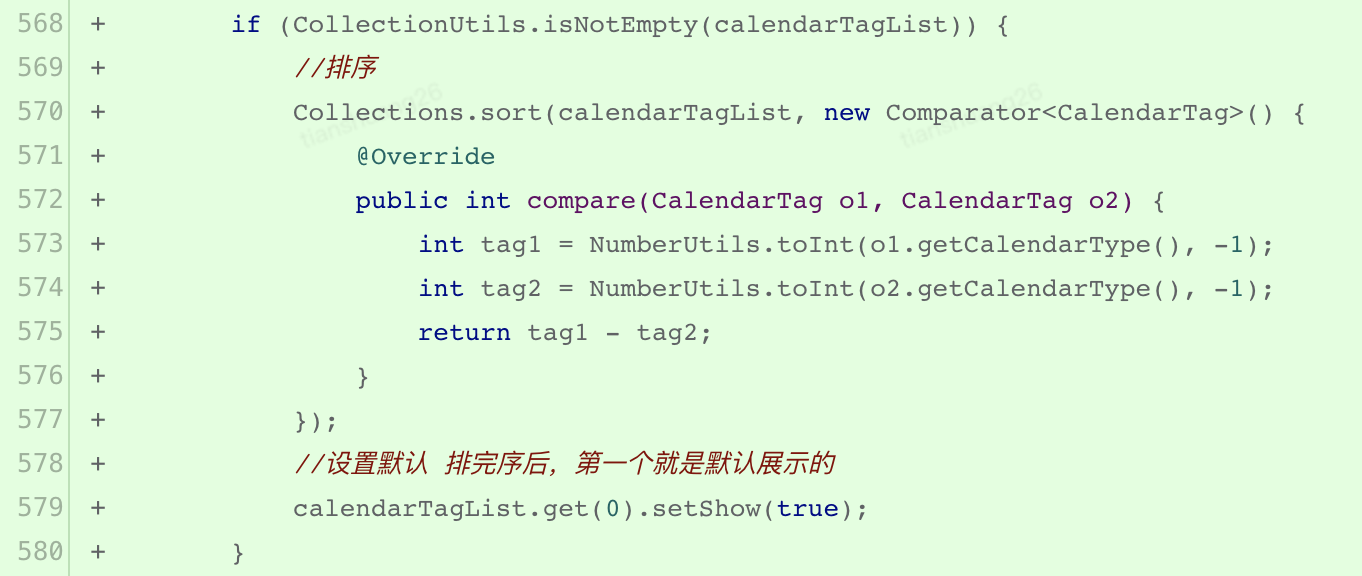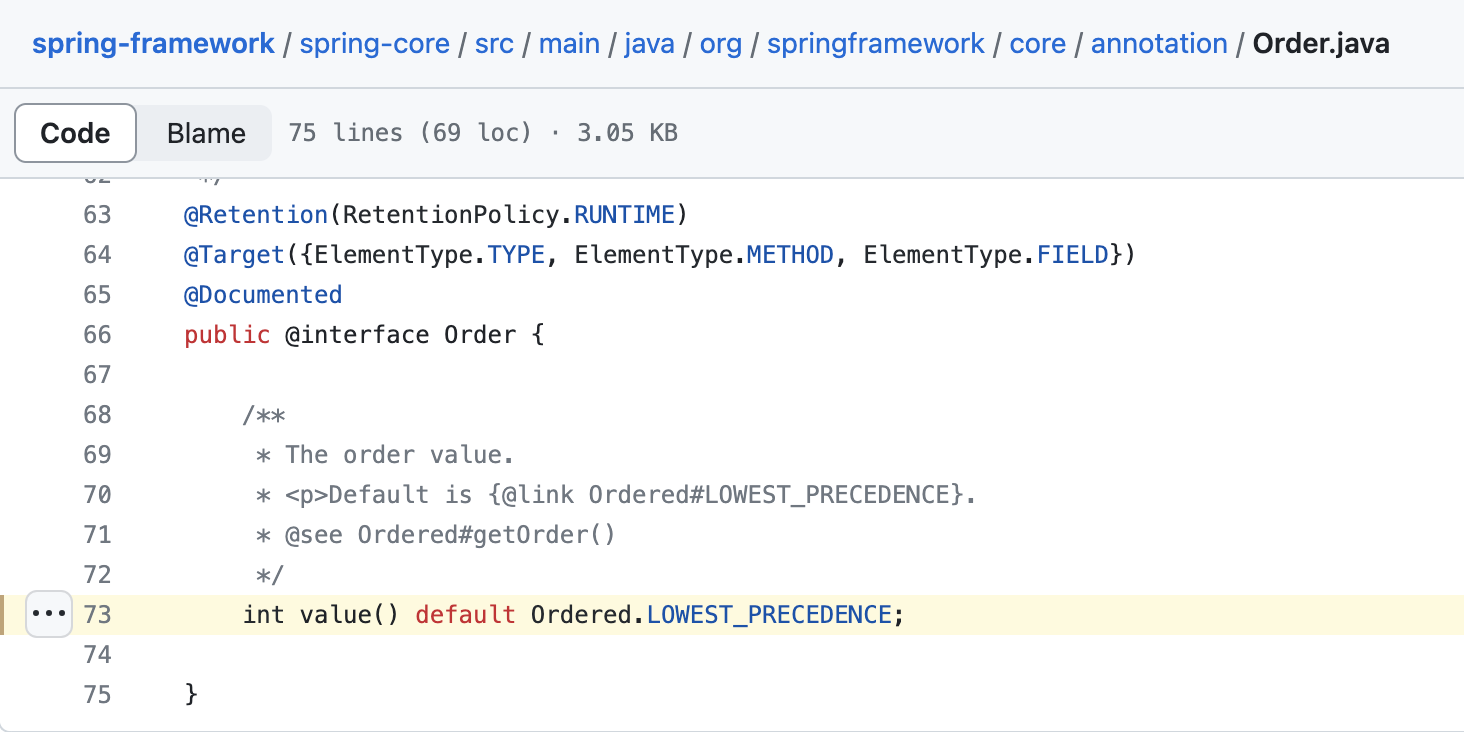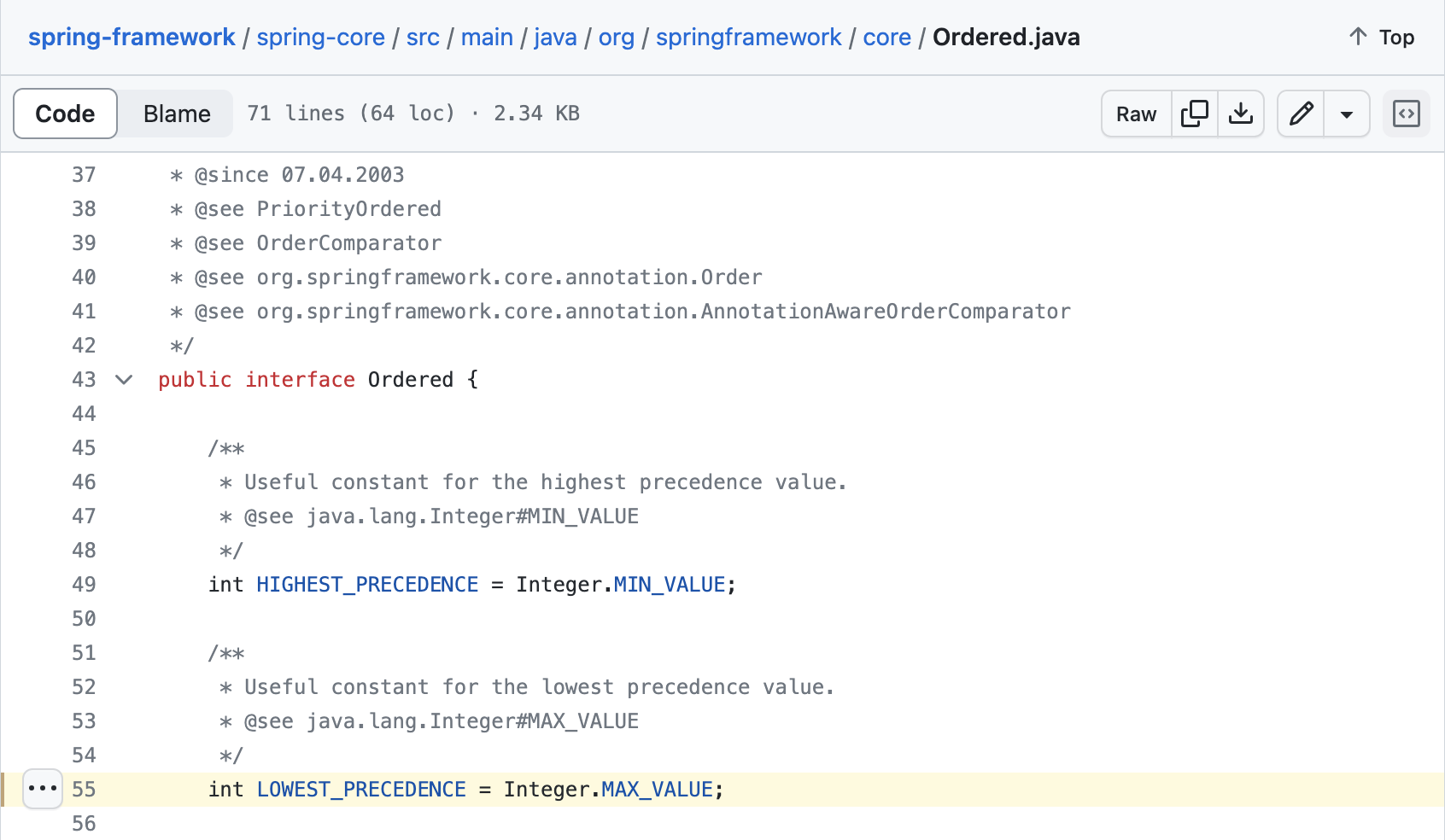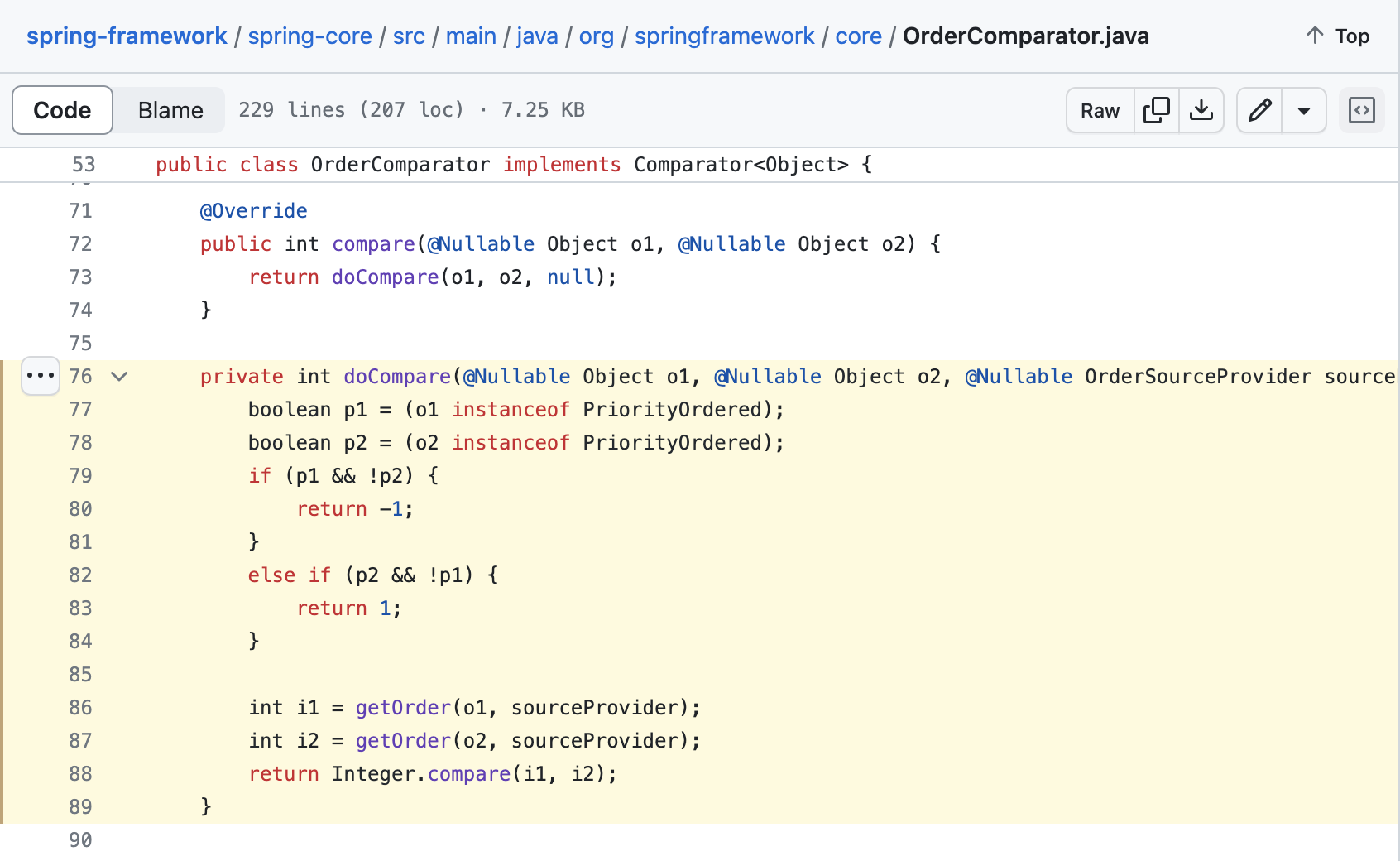/**
* Compares two {@code int} values numerically.
* The value returned is identical to what would be returned by:
* <pre>
*    Integer.valueOf(x).compareTo(Integer.valueOf(y))
* </pre>
*
* @param  x the first {@code int} to compare
* @param  y the second {@code int} to compare
* @return the value {@code 0} if {@code x == y};
*         a value less than {@code 0} if {@code x < y}; and
*         a value greater than {@code 0} if {@code x > y}
* @since 1.7
*/
public static int compare(int x, int y) {
return (x < y) ? -1 : ((x == y) ? 0 : 1);
}



# 切量比例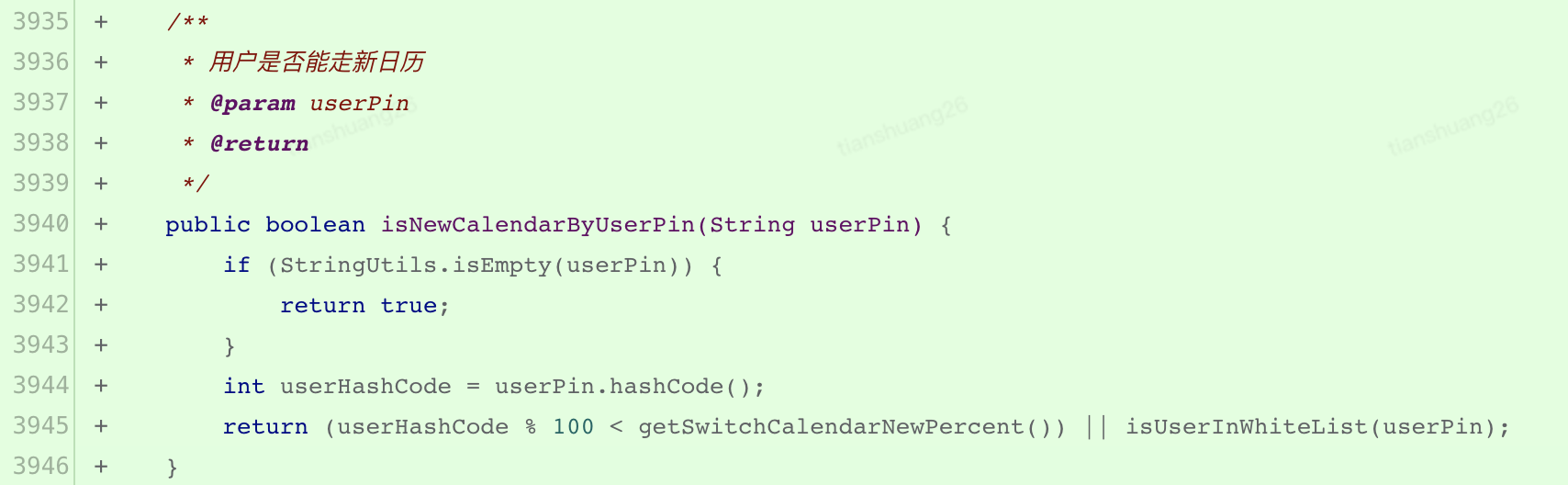/**
* Returns a hash code for this string. The hash code for a
* {@code String} object is computed as
* <blockquote><pre>
* s*31^(n-1) + s*31^(n-2) + ... + s[n-1]
* </pre></blockquote>
* using {@code int} arithmetic, where {@code s[i]} is the
* <i>i</i>th character of the string, {@code n} is the length of
* the string, and {@code ^} indicates exponentiation.
* (The hash value of the empty string is zero.)
*
* @return  a hash code value for this object.
*/
public int hashCode() {
int h = hash;
if (h == 0 && value.length > 0) {
char val[] = value;

for (int i = 0; i < value.length; i++) {
h = 31 * h + val[i];
}
hash = h;
}
return h;
}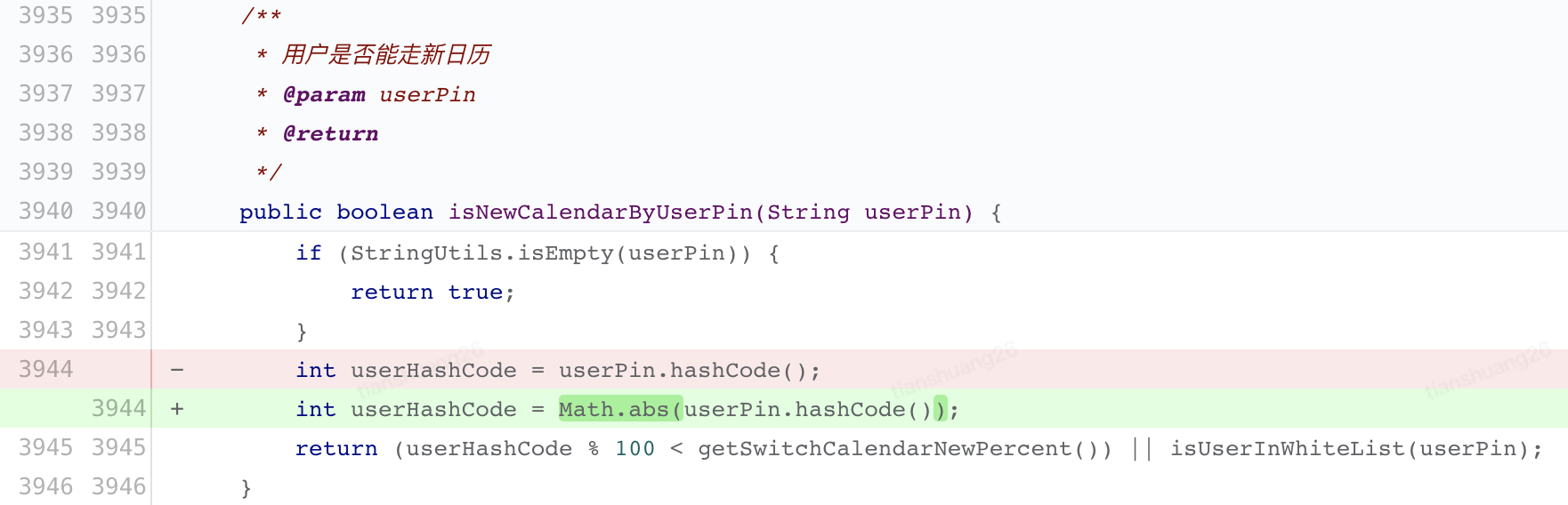/**
* Returns the absolute value of an {@code int} value.
* If the argument is not negative, the argument is returned.
* If the argument is negative, the negation of the argument is returned.
*
* <p>Note that if the argument is equal to the value of
* {@link Integer#MIN_VALUE}, the most negative representable
* {@code int} value, the result is that same value, which is
* negative.
*
* @param   a   the argument whose absolute value is to be determined
* @return  the absolute value of the argument.
*/
public static int abs(int a) {
return (a < 0) ? -a : a;
}



x = Integer.MIN_VALUE:
10000000_00000000_00000000_00000000

~x:
01111111_11111111_11111111_11111111

(~x) + 1:
10000000_00000000_00000000_00000000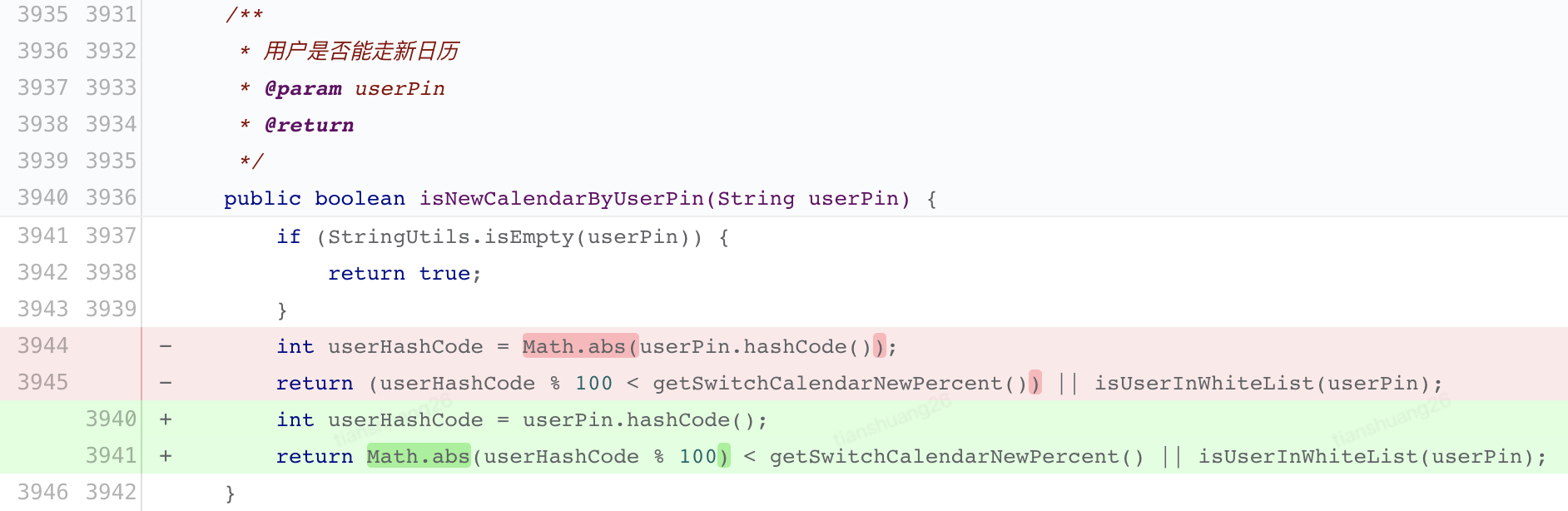# 总结

• java.lang.String#hashCode 在计算过程中可能因为整数越界导致返回值为负数
• Java 语言中的 % 是取余而不是取模，如：(-21) % 4 = (-21) - (-21) / 4 *4 = -1
• Math.abs(int a) 当入参是 Integer.MIN_VALUE 时返回值依然是负数 Integer.MIN_VALUE

# 参考

15.15.4. Unary Minus Operator -

What's the difference between “mod” and “remainder”? - Stack Overflow

Best way to make Java's modulus behave like it should with negative numbers? - Stack Overflow

OrderComparator.java · spring-projects/spring-framework

posted @ 2023-11-20 17:20  京东云开发者  阅读(212)  评论(1编辑  收藏  举报